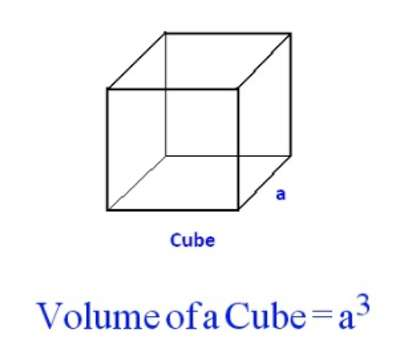Volume of a Cube

A cube is a three-dimensional solid object bounded by six square faces, facets or sides, with three meeting at each vertex.

Volume is the quantity of three-dimensional space enclosed by a closed surface, for example, the space that a substance (solid, liquid, gas, or plasma) or shape occupies or contains. Volume is often quantified numerically using the SI derived unit, the cubic metre. The volume of a container is generally understood to be the capacity of the container; i. e., the amount of fluid (gas or liquid) that the container could hold, rather than the amount of space the container itself displaces.

Volume of a cube = side times side times side. Since each side of a square is the same, it can simply be the length of one side cubed.The volume of a cube is (length of side)3.

The volume of a cube is found by multiplying the length of any edge by itself twice. So if the length of an edge is 4, the volume is- 4 x 4 x 4 = 64

A cube is a unique 3-dimensional shape that has squares for all six of its sides. So, how do we find out how big a cube is? The answer to this question utilizes the object’s volume. The volume of any cube can be determined as the amount of space the cube takes up or as the amount of space inside of the cube.

A cube has six square faces, all of which have sides of equal length and all of which meet at right angles. Finding the volume of a cube is a snap – generally, all that’s needed is to multiply the cube’s length × width × height. Since a cube’s sides are all equal in length, another way of thinking of a cube’s volume is s3, where s is the length of one of the cube’s sides.

Example:

Find the volume of a cube whose edge is 5 cm long.

The length of an edge = 5 cm

Volume of a cube = side of edge × side of edge × side of edge

Volume of a cube = 5 cm × 5 cm × 5 cm = 125 cu cm = 125 cm3.